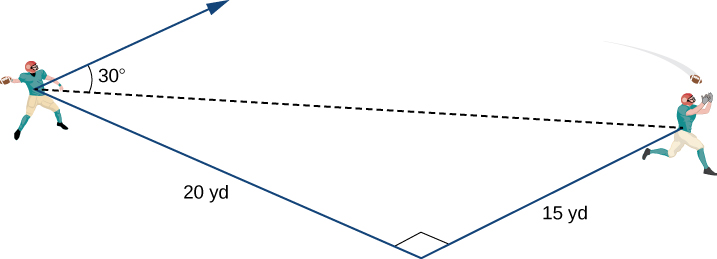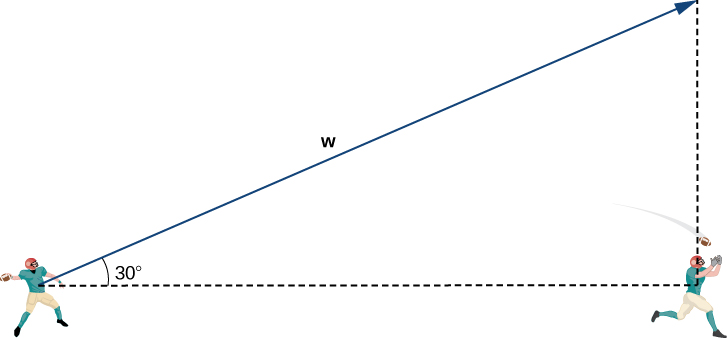# 2.2 Vectors in three dimensions  (Page 6/14)

 Page 6 / 14

Let $\text{v}=⟨-1,-1,1⟩$ and $\text{w}=⟨2,0,1⟩.$ Find a unit vector in the direction of $5\text{v}+3\text{w}.$

$⟨\frac{1}{3\sqrt{10}},-\frac{5}{3\sqrt{10}},\frac{8}{3\sqrt{10}}⟩$

## Throwing a forward pass

A quarterback is standing on the football field preparing to throw a pass. His receiver is standing 20 yd down the field and 15 yd to the quarterback’s left. The quarterback throws the ball at a velocity of 60 mph toward the receiver at an upward angle of $30\text{°}$ (see the following figure). Write the initial velocity vector of the ball, $\text{v},$ in component form.The first thing we want to do is find a vector in the same direction as the velocity vector of the ball. We then scale the vector appropriately so that it has the right magnitude. Consider the vector $\text{w}$ extending from the quarterback’s arm to a point directly above the receiver’s head at an angle of $30\text{°}$ (see the following figure). This vector would have the same direction as $\text{v},$ but it may not have the right magnitude.The receiver is 20 yd down the field and 15 yd to the quarterback’s left. Therefore, the straight-line distance from the quarterback to the receiver is

$\text{Dist from QB to receiver}=\sqrt{{15}^{2}+{20}^{2}}=\sqrt{225+400}=\sqrt{625}=25\phantom{\rule{0.2em}{0ex}}\text{yd}.$

We have $\frac{25}{‖\text{w}‖}=\text{cos}\phantom{\rule{0.2em}{0ex}}30\text{°}.$ Then the magnitude of $\text{w}$ is given by

$‖\text{w}‖=\frac{25}{\text{cos}\phantom{\rule{0.2em}{0ex}}30\text{°}}=\frac{25·2}{\sqrt{3}}=\frac{50}{\sqrt{3}}\phantom{\rule{0.2em}{0ex}}\text{yd}$

and the vertical distance from the receiver to the terminal point of $\text{w}$ is

$\text{Vert dist from receiver to terminal point of}\phantom{\rule{0.2em}{0ex}}\text{w}=‖\text{w}‖\text{sin}\phantom{\rule{0.2em}{0ex}}30\text{°}=\frac{50}{\sqrt{3}}·\frac{1}{2}=\frac{25}{\sqrt{3}}\phantom{\rule{0.2em}{0ex}}\text{yd}.$

Then $\text{w}=⟨20,15,\frac{25}{\sqrt{3}}⟩,$ and has the same direction as $\text{v}.$

Recall, though, that we calculated the magnitude of $\text{w}$ to be $‖\text{w}‖=\frac{50}{\sqrt{3}},$ and $\text{v}$ has magnitude $60$ mph. So, we need to multiply vector $\text{w}$ by an appropriate constant, $k.$ We want to find a value of $k$ so that $‖k\text{w}‖=60$ mph. We have

$‖k\text{w}‖=k‖\text{w}‖=k\frac{50}{\sqrt{3}}\phantom{\rule{0.2em}{0ex}}\text{mph,}$

so we want

$\begin{array}{}\\ \\ \hfill k\frac{50}{\sqrt{3}}& =\hfill & 60\hfill \\ \hfill k& =\hfill & \frac{60\sqrt{3}}{50}\hfill \\ \hfill k& =\hfill & \frac{6\sqrt{3}}{5}.\hfill \end{array}$

Then

$\text{v}=k\text{w}=k⟨20,15,\frac{25}{\sqrt{3}}⟩=\frac{6\sqrt{3}}{5}⟨20,15,\frac{25}{\sqrt{3}}⟩=⟨24\sqrt{3},18\sqrt{3},30⟩.$

Let’s double-check that $‖\text{v}‖=60.$ We have

$‖\text{v}‖=\sqrt{{\left(24\sqrt{3}\right)}^{2}+{\left(18\sqrt{3}\right)}^{2}+{\left(30\right)}^{2}}=\sqrt{1728+972+900}=\sqrt{3600}=60\phantom{\rule{0.2em}{0ex}}\text{mph}.$

So, we have found the correct components for $\text{v}.$

Assume the quarterback and the receiver are in the same place as in the previous example. This time, however, the quarterback throws the ball at velocity of $40$ mph and an angle of $45\text{°}.$ Write the initial velocity vector of the ball, $\text{v},$ in component form.

$\text{v}=⟨16\sqrt{2},12\sqrt{2},20\sqrt{2}⟩$

## Key concepts

• The three-dimensional coordinate system is built around a set of three axes that intersect at right angles at a single point, the origin. Ordered triples $\left(x,y,z\right)$ are used to describe the location of a point in space.
• The distance $d$ between points $\left({x}_{1},{y}_{1},{z}_{1}\right)$ and $\left({x}_{2},{y}_{2},{z}_{2}\right)$ is given by the formula
$d=\sqrt{{\left({x}_{2}-{x}_{1}\right)}^{2}+{\left({y}_{2}-{y}_{1}\right)}^{2}+{\left({z}_{2}-{z}_{1}\right)}^{2}}.$
• In three dimensions, the equations $x=a,y=b,\text{and}\phantom{\rule{0.2em}{0ex}}z=c$ describe planes that are parallel to the coordinate planes.
• The standard equation of a sphere with center $\left(a,b,c\right)$ and radius $r$ is
${\left(x-a\right)}^{2}+{\left(y-b\right)}^{2}+{\left(z-c\right)}^{2}={r}^{2}.$
• In three dimensions, as in two, vectors are commonly expressed in component form, $\text{v}=⟨x,y,z⟩,$ or in terms of the standard unit vectors, $x\text{i}+y\text{j}+z\text{k}.$
• Properties of vectors in space are a natural extension of the properties for vectors in a plane. Let $\text{v}=⟨{x}_{1},{y}_{1},{z}_{1}⟩$ and $\text{w}=⟨{x}_{2},{y}_{2},{z}_{2}⟩$ be vectors, and let $k$ be a scalar.
• Scalar multiplication: $k\text{v}=⟨k{x}_{1},k{y}_{1},k{z}_{1}⟩$
• Vector addition: $\text{v}+\text{w}=⟨{x}_{1},{y}_{1},{z}_{1}⟩+⟨{x}_{2},{y}_{2},{z}_{2}⟩=⟨{x}_{1}+{x}_{2},{y}_{1}+{y}_{2},{z}_{1}+{z}_{2}⟩$
• Vector subtraction: $\text{v}-\text{w}=⟨{x}_{1},{y}_{1},{z}_{1}⟩-⟨{x}_{2},{y}_{2},{z}_{2}⟩=⟨{x}_{1}-{x}_{2},{y}_{1}-{y}_{2},{z}_{1}-{z}_{2}⟩$
• Vector magnitude: $‖\text{v}‖=\sqrt{{x}_{1}{}^{2}+{y}_{1}{}^{2}+{z}_{1}{}^{2}}$
• Unit vector in the direction of v: $\frac{\text{v}}{‖\text{v}‖}=\frac{1}{‖\text{v}‖}⟨{x}_{1},{y}_{1},{z}_{1}⟩=⟨\frac{{x}_{1}}{‖\text{v}‖},\frac{{y}_{1}}{‖\text{v}‖},\frac{{z}_{1}}{‖\text{v}‖}⟩,$ $\text{v}\ne 0$

where we get a research paper on Nano chemistry....?
nanopartical of organic/inorganic / physical chemistry , pdf / thesis / review
Ali
what are the products of Nano chemistry?
There are lots of products of nano chemistry... Like nano coatings.....carbon fiber.. And lots of others..
learn
Even nanotechnology is pretty much all about chemistry... Its the chemistry on quantum or atomic level
learn
da
no nanotechnology is also a part of physics and maths it requires angle formulas and some pressure regarding concepts
Bhagvanji
hey
Giriraj
Preparation and Applications of Nanomaterial for Drug Delivery
revolt
da
Application of nanotechnology in medicine
what is variations in raman spectra for nanomaterials
ya I also want to know the raman spectra
Bhagvanji
I only see partial conversation and what's the question here!
what about nanotechnology for water purification
please someone correct me if I'm wrong but I think one can use nanoparticles, specially silver nanoparticles for water treatment.
Damian
yes that's correct
Professor
I think
Professor
Nasa has use it in the 60's, copper as water purification in the moon travel.
Alexandre
nanocopper obvius
Alexandre
what is the stm
is there industrial application of fullrenes. What is the method to prepare fullrene on large scale.?
Rafiq
industrial application...? mmm I think on the medical side as drug carrier, but you should go deeper on your research, I may be wrong
Damian
How we are making nano material?
what is a peer
What is meant by 'nano scale'?
What is STMs full form?
LITNING
scanning tunneling microscope
Sahil
how nano science is used for hydrophobicity
Santosh
Do u think that Graphene and Fullrene fiber can be used to make Air Plane body structure the lightest and strongest. Rafiq
Rafiq
what is differents between GO and RGO?
Mahi
what is simplest way to understand the applications of nano robots used to detect the cancer affected cell of human body.? How this robot is carried to required site of body cell.? what will be the carrier material and how can be detected that correct delivery of drug is done Rafiq
Rafiq
if virus is killing to make ARTIFICIAL DNA OF GRAPHENE FOR KILLED THE VIRUS .THIS IS OUR ASSUMPTION
Anam
analytical skills graphene is prepared to kill any type viruses .
Anam
Any one who tell me about Preparation and application of Nanomaterial for drug Delivery
Hafiz
what is Nano technology ?
write examples of Nano molecule?
Bob
The nanotechnology is as new science, to scale nanometric
brayan
nanotechnology is the study, desing, synthesis, manipulation and application of materials and functional systems through control of matter at nanoscale
Damian
Is there any normative that regulates the use of silver nanoparticles?
what king of growth are you checking .?
Renato
What fields keep nano created devices from performing or assimulating ? Magnetic fields ? Are do they assimilate ?
why we need to study biomolecules, molecular biology in nanotechnology?
?
Kyle
yes I'm doing my masters in nanotechnology, we are being studying all these domains as well..
why?
what school?
Kyle
biomolecules are e building blocks of every organics and inorganic materials.
Joe
how did you get the value of 2000N.What calculations are needed to arrive at it
Privacy Information Security Software Version 1.1a
Good
can you provide the details of the parametric equations for the lines that defince doubly-ruled surfeces (huperbolids of one sheet and hyperbolic paraboloid). Can you explain each of the variables in the equations?By Anindyo MukhopadhyayBy Madison ChristianBy Dindin SecretoBy Jams KaloBy Robert MurphyBy David GeltnerBy Mary CohenBy Madison ChristianBy Szilárd JankóBy OpenStax